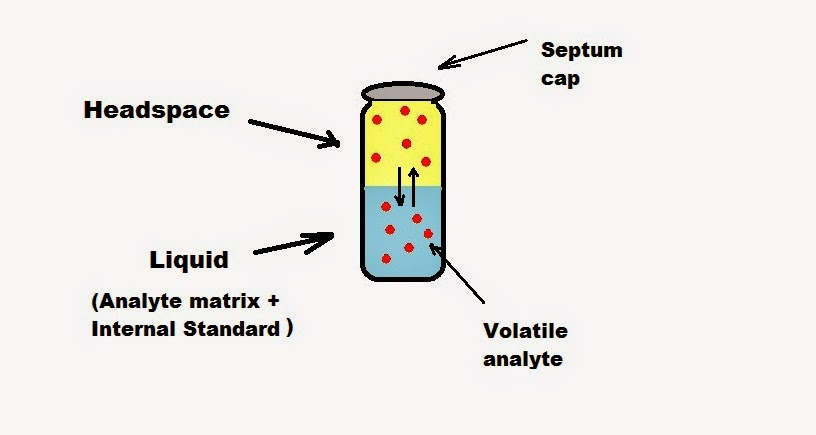Headspace Analysis - Gas Chromatography - Analysis of Ethanol in Blood (Forensic Chemistry) | Chemistry Net

# Headspace Analysis - Gas Chromatography - Analysis of Ethanol in Blood (Forensic Chemistry)

Headspace Analysis - Gas Chromatography - Analysis of Ethanol in Blood (Forensic Chemistry)

Headspace techniques are employed in conjunction with gas chromatography (GC) analysis for certain type of samples. The GC can handle any gaseous sample and any liquid sample that can be vaporized completely and instantaneously before it goes to a proper column for separation. Unfortunately there are many liquid samples (biological, environmental) that cannot be directly injected into the GC. For such samples headspace analysis can be used.
The principle underlying GC headspace analysis is that in a sealed vial at constant temperature equilibrium is established between volatile components of a liquid or solid sample in the vial and the gas phase above it – the “headspace” (Fig. I1). After allowing due time for equilibration (normally 15 min.) a portion of the headspace – ambient volume above a sample matrix where the volatile compounds exist in gaseous form at predictable levels - may be withdrawn via a rubber septum using a gas-tight syringe and injected into the GC column.Fig. I1: GC headspace vial

Headspace analysis is useful for situations where:
• The analyte of interest is volatile at temperatures below 290 C
• The sample matrix is a solid, liquid, paste that is not easy to inject into a GC inlet
• Sample preparation to allow easy liquid injection is difficult

The following video demonstrates the headspace sampler of a GC-FID system:

• Simpler sample preparation
• Directly analyze a wide range of sample matrices such as liquids, solids and pastes
• Columns last longer, with less maintenance. The headspace volume above the sample matrix is more clean than the matrix. By injecting fewer contaminants the analytical column lasts longer.
• High precision
• Solvent peak is smaller or nonexistent compared to traditional liquid injection GC techniques.

An internal standard may be added prior to the heating process, and quantitative analyses may be performed after constructing a calibration graph.
This technique is widely used in the analysis of ethanol in blood (blood-alcohol in driving under influence (DUI) and driving whil and other volatile substances in biological samples and in the pharmaceutical industry for measuring solvent residues in tablets, amongst other applications. A gas chromatogram of an in-house reference material containing ethanol, methanol and n-propanol obtained by using headspace analysis is shown in Fig. I2.Fig. I2: A gas chromatogram of an in-house reference material containing ethanol, methanol and n-propanol obtained by using headspace analysis is shown above. The first peak is acetaldehyde (retention time: 0.886 min), the second peak is ethanol (retention time: 1.407 min) and the last peak is isopropanol the internal standard (retention time: 3.070 min)

The equations describing headspace theory derive from three physical laws associated with vapor pressure, partial pressures , and the relationship between vapor pressure of an analyte above a solution and the concentration of that analyte in the solution. These laws are: Dalton’s law, Henry’s law and Raoult’s law
According to the above laws the basic principle involved in headspace theory is the establishment of equilibrium between the sample phase and the headspace above the sample. In a vial, at the end of the equilibration process, the concentration of sample analyte in the headspace volume is given by the mass balance:

CO * VL = CG * VG + CL * VL        (1)
Where:
CO is the concentration of analyte in the original sample (mol/ml)
CG is the equilibrium concentration of analyte in the headspace (mol/cm3)
CL is the equilibrium concentration of analyte in the liquid phase (mol/ml)
VL is the volume of the sample (cm3)
VG is the volume of gas in the sample vial (cm3)

Since the distribution coefficient (Henry’s law constant) is defined as:

H = CL / CG         (2)

By combining equations (1) and (2) and rearranging:

1/ CG  = (H/ CO) + (1/ CO) * (VG / CL)        (3)

In most cases at a low concentration range, the GC area count is directly proportional to the gas phase concentration (CG). Equation (3) can be rewritten as:

1/ A  = (H*Rf/ CO) + (Rf / CO) * (VG / CL)        (4)

where A is the GC area count and Rf is the GC response factor.

A plot of the inverse of the GC area count (1/A) versus (VG / CL) results in a straight line, as predicted by equation (4). The original concentration, CO, can be obtained from the slope of the straight line, while the dimensionless Henry’s law constant (H) can  be calculated as the ratio of intercept to slope.

#### References

“Applied Headspace Gas Chromatography”, B. Kolb Editions, Heyden, London, 1980
M. Markelov et al., Anal. Chim. Acta, 276, 235-45, (1993)
T. Podar, J. of Chrom. Science, 35, 565 – 567, (1997)
R. J. Flanagan et al. “Fundamentals of Analytical Toxicology”, John Wiley & Sons Ltd., 2007

#### 1 comment:

1.Can you please provide a method for ethanol detection in blood ? We are using agilent hs gc ..Which perameters we have to use and how much amount of blood and internal standard add in vial ?. How calibration graph perform